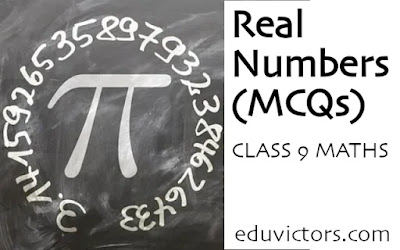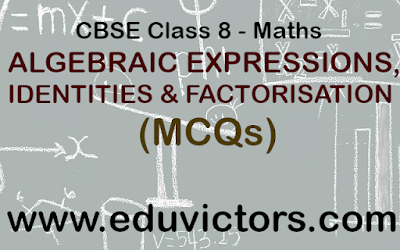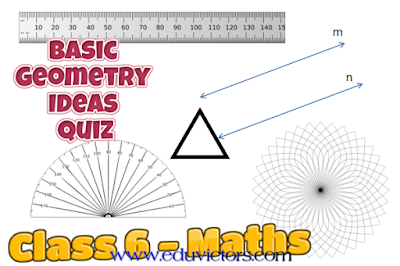## Pages

Showing posts with label maths-quiz. Show all posts
Showing posts with label maths-quiz. Show all posts

# CBSE Class 9 - Maths - Real Numbers (MCQs)

Choose the correct option in each of the following questions:

Q1. Which of the following is a rational number?

(a) 2√3

(b) π

(c) 0

(d) √3 + 1

Q2. Every point on a number line represents

(a) a rational number

(b) a natural number

(c) an irrational number

(d) a real number# CBSE Class 10 Maths Revision Worksheet (All Chapters)

Fill in the blanks with suitable words.

1. HCF of any two primes numbers is always __________.

2. LCM of Smallest prime number and the smallest composite number is _______.

3. A _________ is a polynomial of degree 0.

4. We get the original polynomial if we multiply its ________ together.

5. If we draw x = a and y = b graphically then we get lines intersecting at _________.## ALGEBRAIC EXPRESSIONS, IDENTITIES AND FACTORISATION

(MCQs)

Q1: Which is the like term as 24a²bc?
(a) 13 × 8a × 2b × c × a
(b) 8 × 3 × a × b × c
(c) 3 × 8 × a × b × c × c
(d) 3 × 8 × a × b × b × c## Basic Geometry Ideas - Quiz

Class 6 - Mathematics

Q1: Three or more lines which pass through same point are called

(a) intersecting lines
(b) parallel lines
(c) perpendicular lines
(d) concurrent lines.

Q2: Geometrical figure which has no dimension is

(a) line
(b) plane
(c) line segment
(d) point.

Q3: The lines which do not intersect and have equal distance between them are called:

(a) parallel lines
(b) perpendicular lines
(c) intersecting lines
(d) straight lines